# Originally given April 14, 1992

Note: This is for practice only. The actual exam will not cover random variables (problems 4 and 5). For extra practice on combinatorics, look at the practice first exams. You may want to save problems 4 and 5 to help you practice for the third exam.

Problem 1: A baseball team needs to win 4 (or more) of their last 6 games to win the pennant. Assuming they're a good team team, and have a 60% chance of winning any given game, what is the probability of their winning the pennant?

Problem 2: There are 5 blue balls, 3 red balls and one white ball in an urn.

a) If you pick 4 balls without replacement, what is the probability of drawing (exactly) 2 blue balls?

b) If you draw 4 balls WITH replacement, what is the probability of drawing (exactly) 2 blue balls?

Problem 3: A standard bridge hand consists of 13 cards. a) What is the probability of being dealt a hand with exactly 4 spades, 4 hearts and 4 diamonds (and one club)? b) What is the probability of being dealt 4 spades OR 4 hearts OR 4 diamonds? (For both parts, write down an exact expression but do not attempt to simplity).

Problem 4: X and Y are discrete random variables. Their joint probability density function is: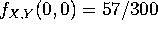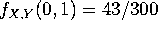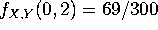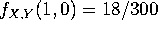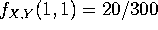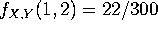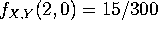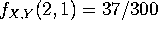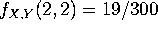. a) Compute the marginal probability densities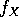and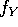. b) Are the variables X and Y independent? c) What is the (conditional) probability that X=2 given that Y=1? d) Are the events X=1 and Y=0 independent?

Problem 5: a) X and Y are continuous random variables with the joint density function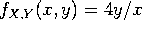if 0 < y < x and 0 otherwise. Compute the density function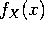and the probability that X < 0.4. b) Let Z be a continuous random variable with density function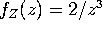for z > 1. Compute the cumulative distribution function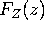and the mean E(Z).

# Solutions

Problem 1: The number of games won is given by a binomial distribution with n=6 and p=0.6. We care about k=4,5,6. The answer is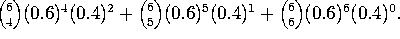Problem 2a. This is hypergeometric. There are 5 blue balls and 4 non-blue balls, so the answer is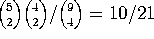. You can also get the same answer by adding the probability of (2 blue, 1 white, 1 red) to the probability of (2 blue, 2 red).

Problem 2b. This is binomial.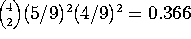.

Problem 3a. You must choose 4 of the 13 spades, 4 of the 13 hearts, 4 of the 13 diamonds, and 1 of the 13 clubs. The number of ways of doing this is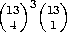. Dividing by the number of hands,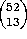, we get our answer.

Problem 3b. By the inclusion/exclusion principle, P(4S OR 4H OR 4D) = P(4S)+ P(4H) + P(4D) - P(4S AND 4H) - P(4S AND 4D) - P(4H AND 4D) + P(4S AND 4H AND 4D) = 3P(4S) - 3P(4S AND 4H) + P(4S AND 4H AND 4D), which totals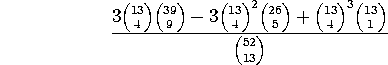Problem 4. a. Adding rows and columns we get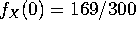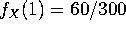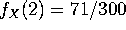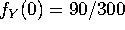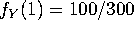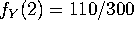.

b. No. Since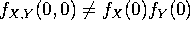, (one of many possible examples), X and Y are not independent.

c.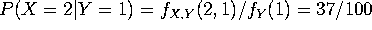.

d. Since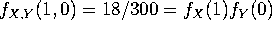, the two events ARE independent.

Problem 5a.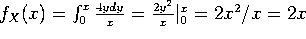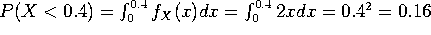.

5b.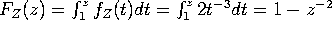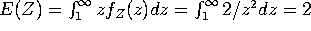.